# Reverse a Linked List in C++: Iterative & Recursive (with code)

Many of you must be familiar with the application of a linked list in the real world and its importance. We use linked lists to maintain a directory of names, dynamic allocation of memory, and create an implementation of essential data structures like stacks and queues, and whatnot. In this article, we will learn how to reverse a linked list in C++. Let’s get Kraken!

## What is a Linked List in C++?

A linked list is a sequence of links that contain elements connected using pointers. Each link which is a pointer contains a connection to another node.  The last node has a null pointer to indicate it is the end. The linked list is the second most utilized data structure after arrays.

There are three components of a linked list:

1. Node: Each node stores data which is called an element.
2. Next: Every node of a linked list contains a link to the next link that is Next.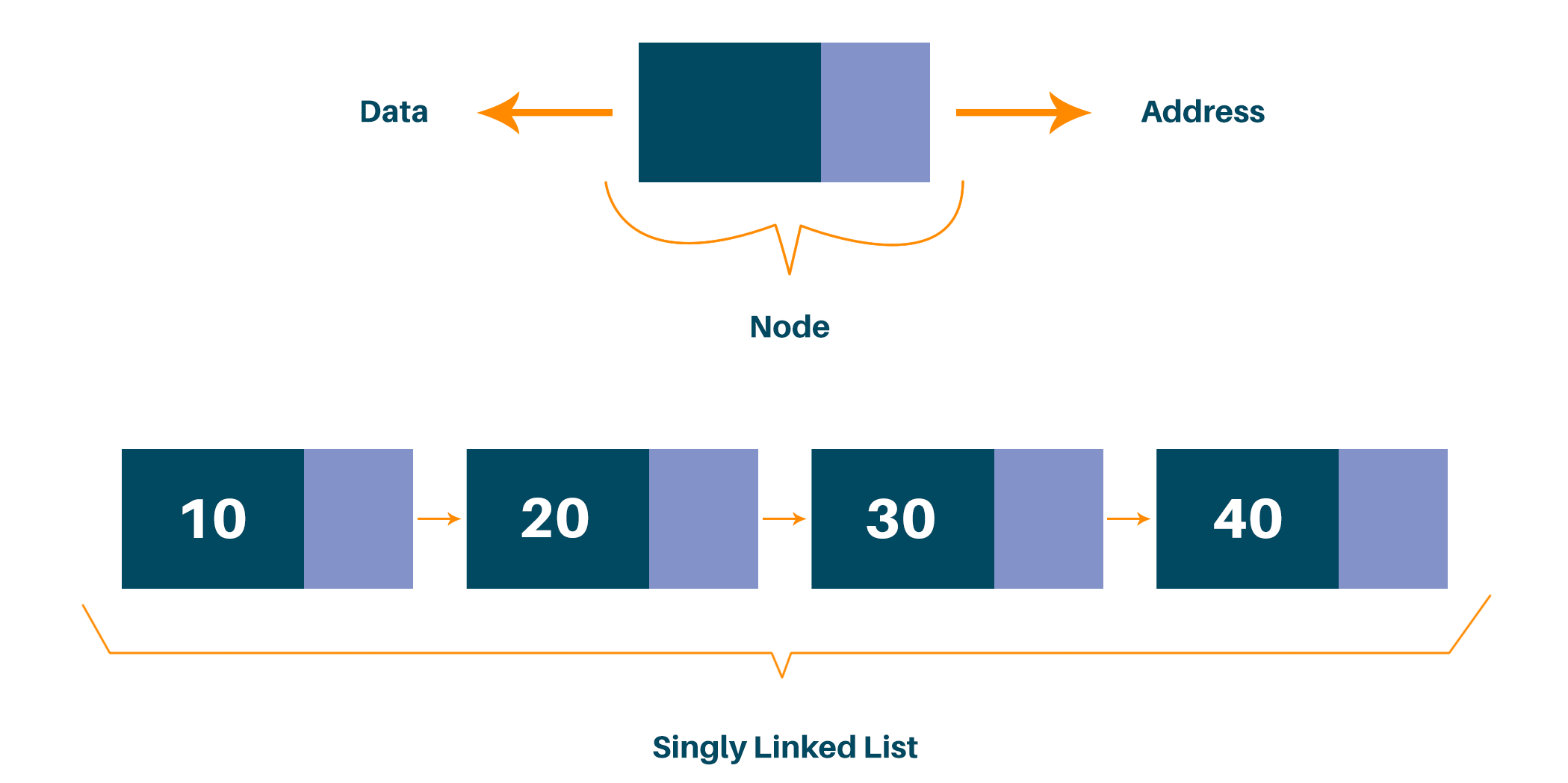## How to Reverse a Linked List in C++?

Let's understand the problem statement first to clearly understand what we are going to do.

Reversing the linked list implies reversing all the elements and we can do it by reversing all the links and making the next pointer point to the previous node. In this way, the last node becomes the first node, the second-to-last node becomes the second node, and so on.

Here, we are provided with the pointer or reference to the head of a singly linked list, invert the list, and return the pointer or reference to the head of the new reversed linked list. For example, consider the following linked list: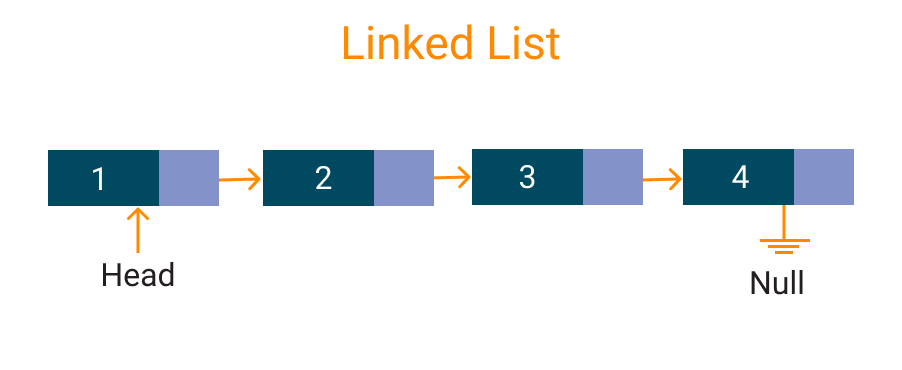After reversing the complete linked list we return the pointer to the new linked list as demonstrated in the figure: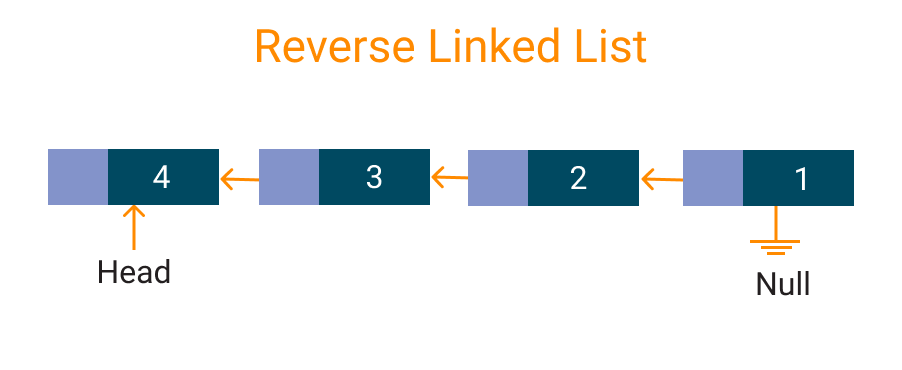There can be two approaches to solving this problem, the following are the hints, try solving the problem by yourself, and then head over to the next section to find the solution and C++ code.

There are mainly two approaches to implementing it in C++:

1. Think of an iterative approach to find the reversed list in a single pass.
2. Or, think of a recursive approach to find the reversed list in a single pass.

Now that you have tried solving the problem yourself given the two types of approaches to solving this problem, let’s discuss both approaches one by one:

### Iterative Solution for Reversing a Linked List in C++

If the linked list has only one or no element, then we return the current list as it is. And if there are two or more elements, then we can implement an iterative solution using three-pointers

We will create a function to reverse the linked list taking reference to the head node and as the only argument and return the head of the new linked list:

• Step 1: Define three nodes one with the reference to the head node and name it current, and name the other two nodes temp and prev pointers as NULL.
• Step 2: Using a while loop we will traverse the linked list once until the next pointer does not become NULL.
• Step 3: While iterating, we perform the following operations:

temp = current->next;

current->next = prev;

prev = current;

current = temp;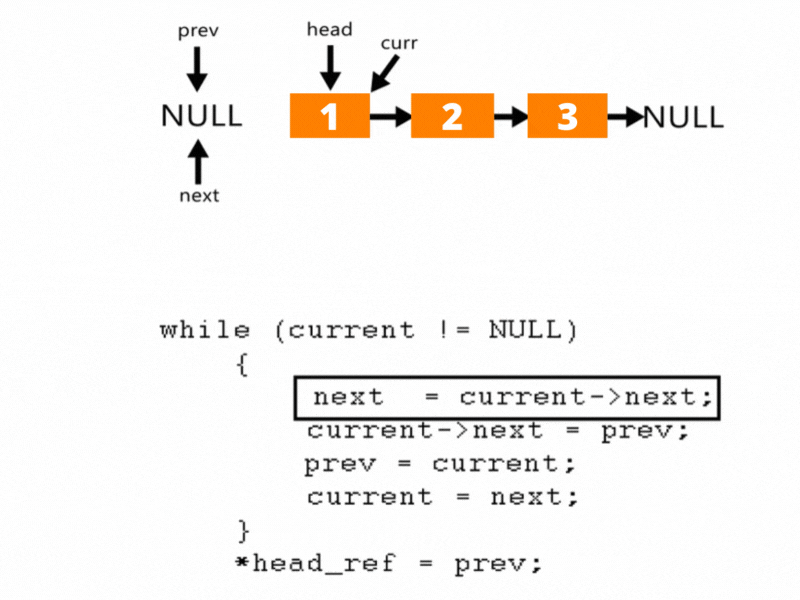We assign the temp node to the next of the current node and then reverse the link by assigning the current->next to the previous node. And then increment the previous node to the current node and then the current node to the temp node.  And then we finally return the head node.

The full C++ code for the iterative implementation of reversing a linked list  is given below:

```#include<bits/stdc++.h>

using namespace std;

struct node {
int data;
struct node *next;
};

// To create a demo we have to construct a linked list and this
// function is to push the elements to the list.
void push(struct node **head_ref, int data) {
struct node *node;
node = (struct node*)malloc(sizeof(struct node));
node->data = data;
}

// Function to reverse the list
struct node *temp = NULL;
struct node *prev = NULL;
while(current != NULL) {
temp = current->next;
current->next = prev;
prev = current;
current = temp;
}
}

// To check our program
}
}

// Driver function
int main() {
cout << "Linked List Before Reversing" << endl;
cout << endl;
cout << "Linked List After Reversing"<<endl;
return 0;
}
```

Output:

```Linked List Before Reversing

10 4 0 8 1 0

0 1 8 0 4 10
```

Time & Space complexity: The time complexity is O(N) because we iterate through each element at least once. The space complexity is O(1) because no extra space was used here.

### Recursive Solution for Reversing a Linked List in C++

The most important thing to remember in this approach is that the recursive approach uses a stack. The compiler allocates stack memory after each recursive call, and this solution can run out of memory in case of very huge linked lists (think billions of elements).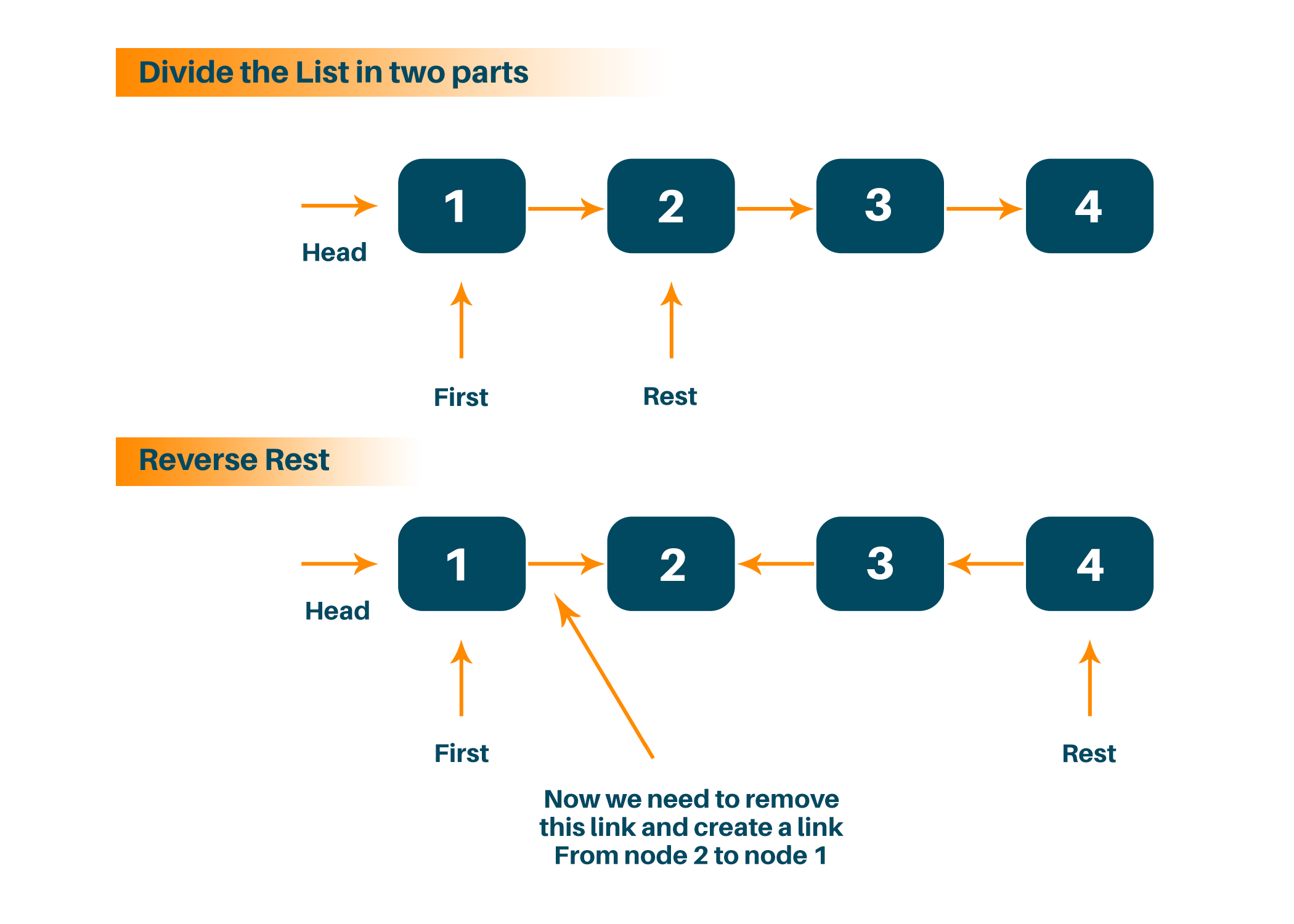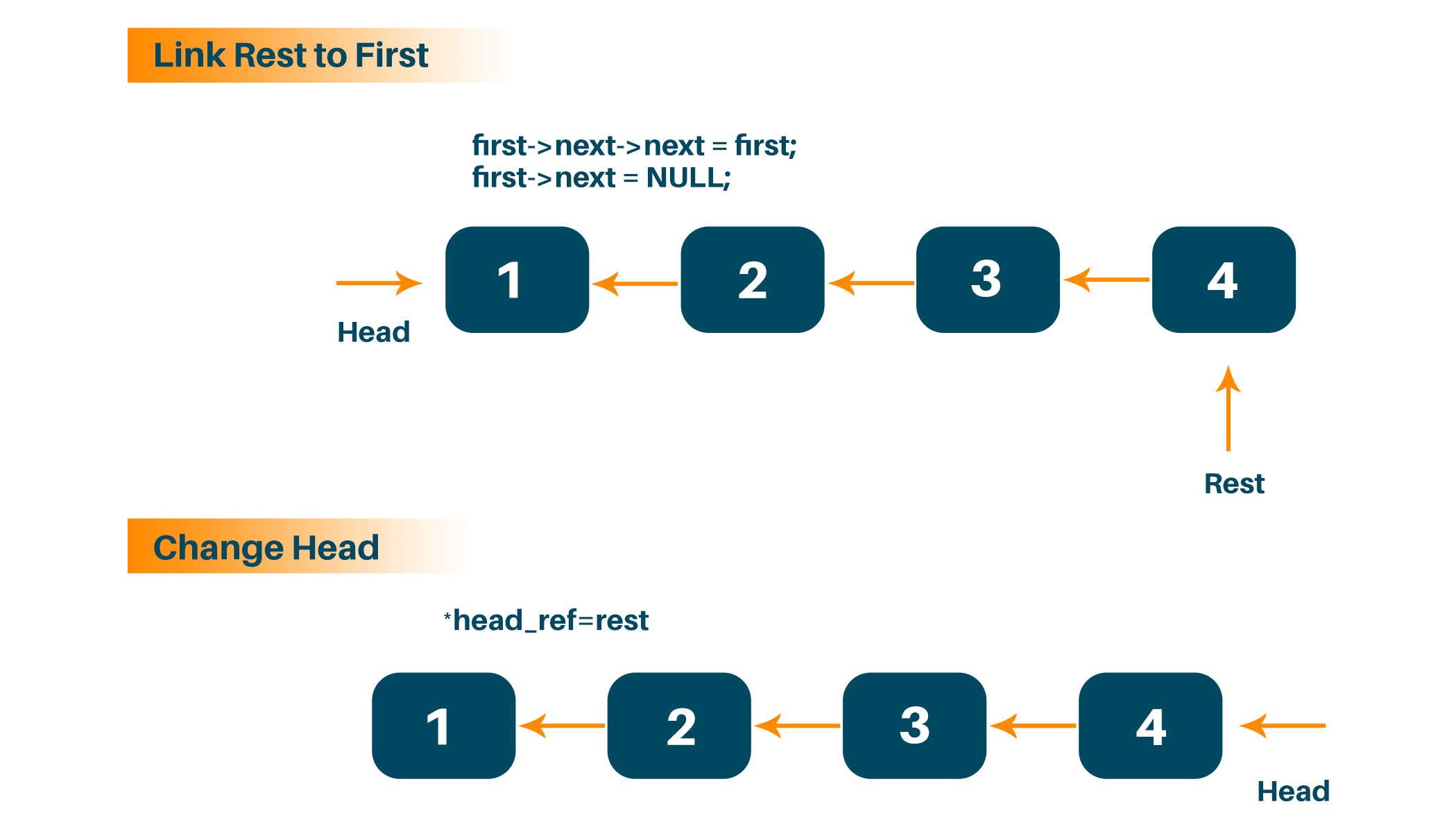We recursively iterate to each node in the list until we reach the last node and return the new head. We have to note that the last node in this approach will become the new head of the list. On the return path, each node is going to append itself to the end of the partially reversed linked list.

The recursive implementation of reversing a linked list in C++ is as follow:

```#include<bits/stdc++.h>
using namespace std;

struct Node {
int data;
struct Node* next;
Node(int data)
{
this->data = data;
next = NULL;
}
};

{
}

{
// Recursive call

return rest;
}

void print()
{
while (temp != NULL) {
cout << temp->data << " ";
temp = temp->next;
}
}

void push(int data)
{
Node* temp = new Node(data);
}
};

int main()
{
ll.push(320);
ll.push(34);
ll.push(315);
ll.push(385);

cout << "Linked List Before Reversing\n";
ll.print();

cout << "\nLinked List After Reversing \n";
ll.print();
return 0;
}
```

Output:

```Linked List Before Reversing

385 315 34 320

320 34 315 385
```

Time & Space Complexity: The time complexity is O(N) because we iterate through each element at least once. The space complexity is O(N) because we create a recursive stack each time we call the reverse function recursively.

### Reverse a Linked List by Tail Recursive Method in C++

A linked list is a type of data structure where a series of nodes are connected by pointers. A node is composed of data plus a pointer to the following node in the chain.  Recursively traversing the list and changing the pointers as you go allows you to reverse a linked list using the tail recursive approach.

Despite requiring a helper function to send the list's tail to the recursion, the tail recursive approach is effective and has an O(1) space complexity. The tail recursive method is a technique that uses a recursive function where the recursive call is the last operation performed by the function.

The complete code is given below:

```#include <iostream>

struct Node {
int data;
Node* next;
};

Node* Reverse(Node* current, Node* previous) {
if (current == nullptr) {
return previous;
}
Node* next = current->next;
current->next = previous;
return Reverse(next, current);
}

}

int main() {
// create a linked list with 5 nodes

std::cout << "Original linked list: ";
while (current != nullptr) {
std::cout << current->data << " ";
current = current->next;
}
std::cout << std::endl;

std::cout << "Reversed linked list: ";
while (current != nullptr) {
std::cout << current->data << " ";
current = current->next;
}
std::cout << std::endl;

// free the memory allocated for the linked list
while (current != nullptr) {
Node* temp = current;
current = current->next;
delete temp;
}

return 0;
}```

A node in a linked list is represented by a Node struct, which is defined in the code above. A linked list is reversed using tail recursion by scanning the list repeatedly and updating each node's next pointer. The ReverseList method is a wrapper that makes an initial call to Reverse with a prior set to nullptr.

By building a linked list with 5 nodes, using the ReverseList function to flip the list, and reporting both the original and reversed lists to the console, the main function shows how to utilize the function. The code then deletes each node in turn, iterating over the list and freeing the memory allotted for the linked list.

## Conclusion

In this tutorial, we learned how to reverse a linked list in C++ using two approaches, and discussed the code in C++. This question is frequently asked in technical interviews by huge companies like Amazon and Google. Happy Learning :)

### FavTutor - 24x7 Live Coding Help from Expert Tutors!